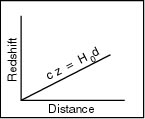When you made graphed magnitude vs. redshift in the last section, you went through steps similar to the ones Edwin Hubble went through in 1929. Now, you are at the same point Hubble was at in 1929: you must interpret your diagram to learn something useful about the universe.

Hubble made two other important observations that led him from the straight line he saw in his data to the picture of the expanding universe. First, the line in his distance-redshift diagram does not depend on direction in the sky – in one direction we see redshifts, as if galaxies are moving away from us, and in the opposite direction we also see redshifts, not blueshifts. Everywhere, galaxies are moving away from us, and the farther they are, the faster they move.

Second, counts of galaxies in various directions in the sky, and to various distances, suggest that space is uniformly filled with galaxies. (Although galaxies cluster, the clusters average out over all of space).

## The Center of the Universe?

Since we see all galaxies moving away from us, you might conclude that we are at the center of the universe. But this is not the case. Think back to the balloon – each point on the surface of the balloon got farther away from every other point, but no point was at the “center” of the balloon. All points move away from each other because of the expansion of the balloon’s surface.

Similarly, all galaxies in the universe move away from one another because of the expansion of space in the universe. There is no “center” to the universe – we would see the same straight line on the Hubble Diagram if we were observing from any other galaxy. Galaxies move away from each other because of the expansion of the universe.

Question 4: You now know that we are not at the center of the universe – that we would see a straight line in the Hubble diagram from any place in the universe. So how does the straight line imply that the universe is expanding?

### The Equation for the LineThe line on the Hubble diagram that relates redshift to distance is expressed as

c z = H0 d ,

where c is the speed of light, z is the redshift, d is the distance, and H0 is called the Hubble constant. The value of H0 depends on the units used to measure the distance d. The subscript 0 tells us “measured at the present time,” which suggests that its value may have been different earlier.

If you were to ask an astronomer what the distance to some particular galaxy was, most likely she or he would measure the redshift z and use the formula above to compute d. This is not what you did: you were trying to establish that the formula itself was right, which means that you had to estimate d independently from your measurement of redshift. But assuming the universe is expanding, the equation above provides an easy way to measure the distance to any galaxy – as long as you know the value of H0, the Hubble constant.